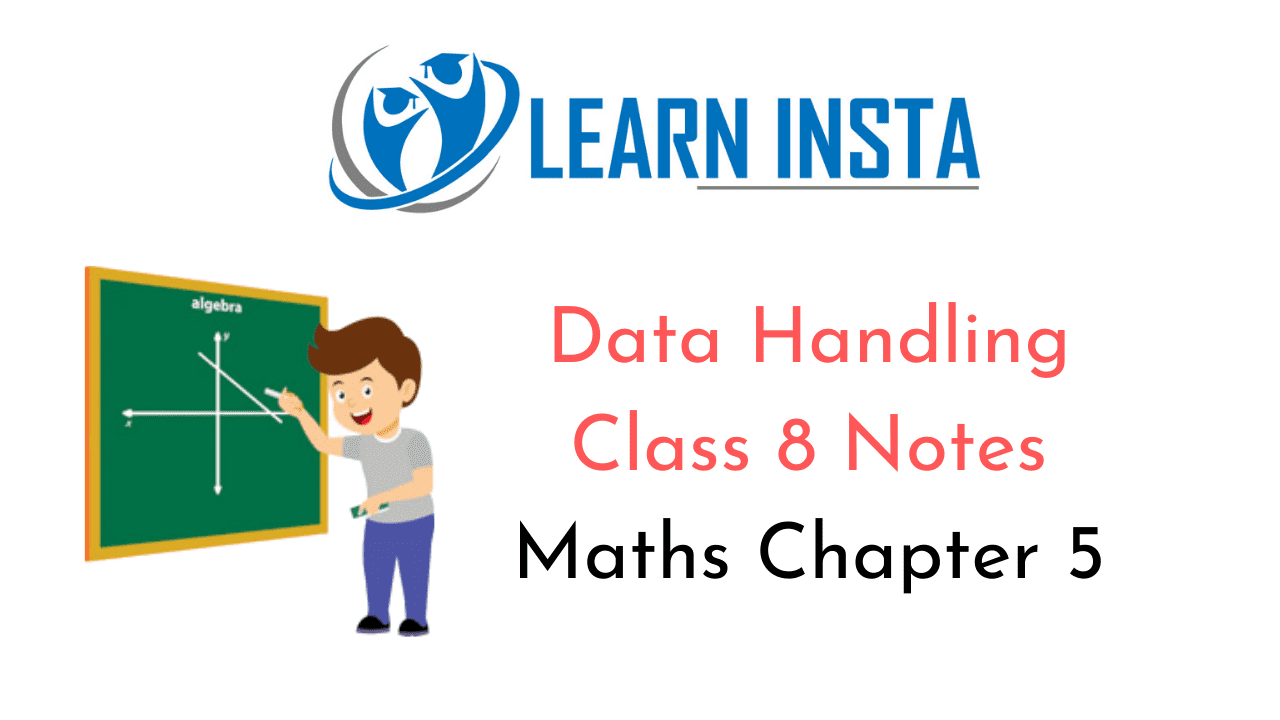On this page, you will find Data Handling Class 8 Notes Maths Chapter 5 Pdf free download. CBSE NCERT Class 8 Maths Notes Chapter 5 Data Handling will seemingly help them to revise the important concepts in less time.

## CBSE Class 8 Maths Chapter 5 Notes Data Handling

### Data Handling Class 8 Notes Conceptual Facts

1. Data: Collection of information as numerical facts about the objects or events is called Data.

2. Types of Data: Discrete and continuous.

3. Frequency: The number of times each score occurs is called frequency.

4. Range: The difference between the greatest and the least observations is called the Range.

5. Relative Frequency: The ratio of the frequency of each item to the sum of all frequencies is called Relative frequency.
$$\text { Relative frequency }=\frac{\text { Frequency of an object }}{\text { Total frequency }}$$

6. Class-size: Difference between upper and lower limits of a class interval is called class-size.

7. Class-mark: Mid value of class interval is called its class-mark.
$$\text { Class-mark }=\frac{\text { Upper limit }+\text { Lower limit }}{2}$$

8. Class-frequency: The frequency of a particular class-interval is called class-frequency.

9. Bar Graph: Horizontal and vertical Histogram: Horizontal and vertical

10. Pie chart: Pie chart is a way of representing the data in the form of sectors of a circle.
Central angle for a sector = $$\left(\frac{\text { Value of the component }}{\text { Total value }} \times 360\right)^{\circ}$$